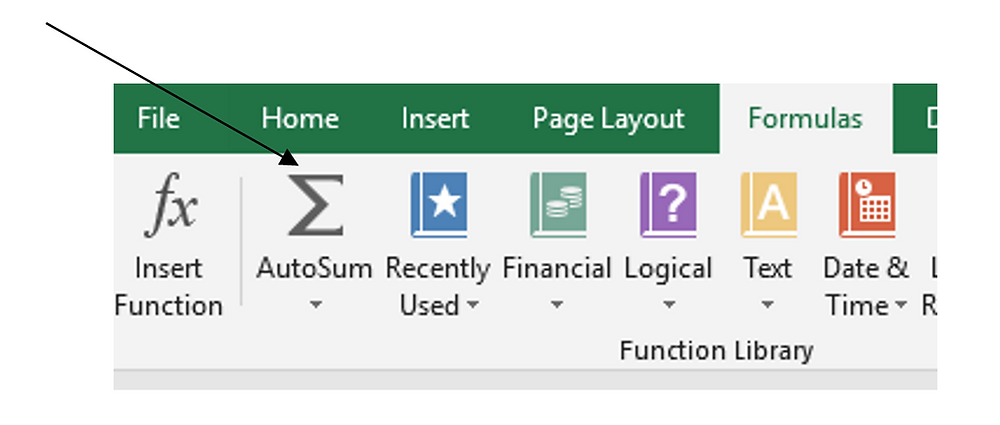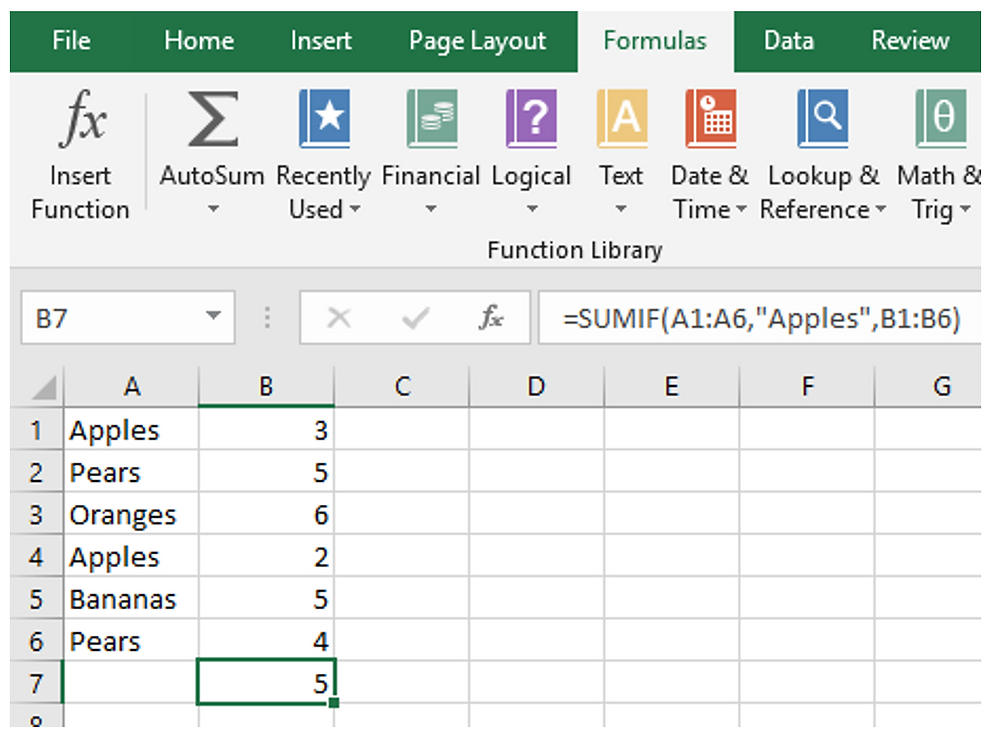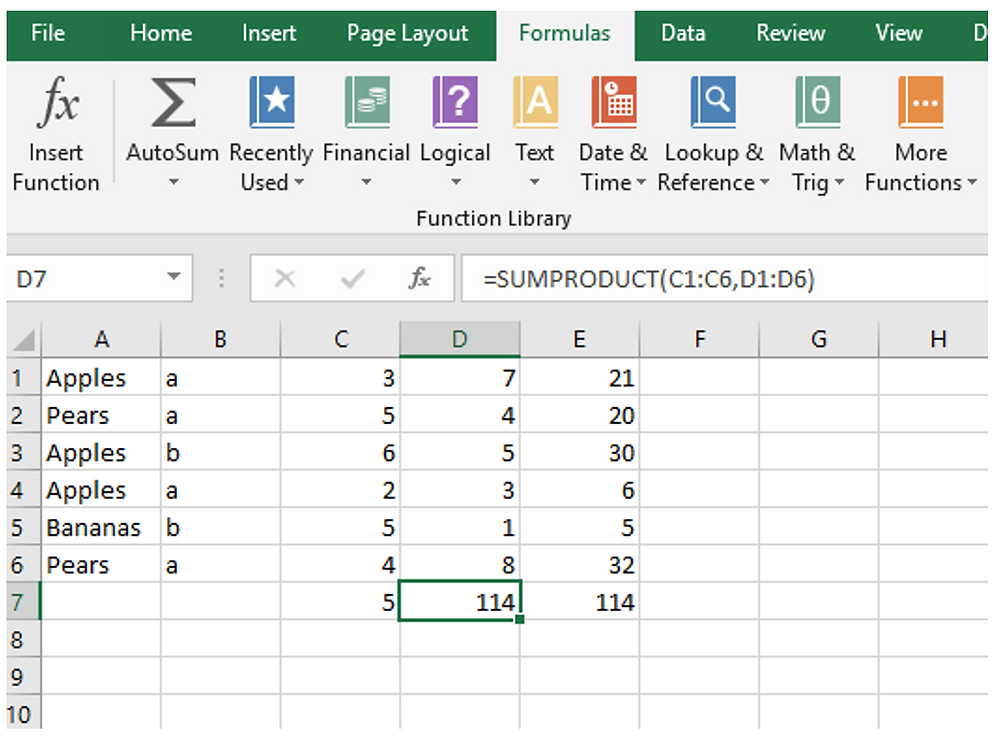Search
• Got it Pass Team

# Most Common Excel Functions: MS Excel 2019 Functions in Accounting

Updated: Sep 30, 2020

Mastering most common excel functions is definitely helpful in your number crunching jobs. In this article, we cover basic to advance functions that are commonly used in accounting, such as summing functions, counting functions, loan repayment function and more.

In addition, we share tips and tricks to you on how to deal with complicated formula to improve your efficiency.

## Summing Functions

In Excel, the summing functions are the most widely used, particularly for accountants. These are the main sum functions that accountants will use:

SUM – this is the most straight forward of the sum functions and it simply totals a range of cells e.g. =SUM (A1:A10)

A shortcut for this is the AutoSum feature. Select the cell at the end of the column/row of numbers and click on AutoSum (Formula tab of ribbon, Function Library group). The sum formula will be inserted based on the numbers in the column / row.MS Excel Function - AutoSum feature

SUMIF – this function allows you to put in criteria for what numbers you want to be included in the result. In the example shown, column A has a description and column B has numbers

If you want to sum the rows with a description of ‘Apples’ then you use =SUMIF (A1:A6,” Apples”, B1:B6)MS Excel Function - SumIf feature

The function requires three parameters.

1. The range holding the description

2. The criteria. Note that the text is in quotes. You can also use wildcards here, so ‘A*’ would produce the same result as ‘Apples’. You can also use parameters like ‘<>’ to get the sum of everything except apples.

3. The range holding the numbers to be summated

SUMIFS – this function allows you to enter extra parameters as your criteria. Effectively, it is like an ‘AND’ statement in a database query.

In this example, there is an extra column (B) which has additional data against the data in column A

Note that the letters in column B do not relate to the description in column A. To sum the total for apples which have a value of ‘a’ in column B the function would be:

=SUMIFS (C1:C6, A1:A6,"Apples”, B1:B6,"a")MS Excel Function - SumIfs feature

This would add C1 + C4, but would not include C3 as that has a value of ‘b’ in B3

As for SUMIF, you can use wildcards and ‘<>’

SUMPRODUCT – this function takes two adjacent ranges, multiplies the related numbers together, and sums the result.

In the example, column D holds another range of numbers, which could be pricing. To get the total of the product of column C and column D you use:

=SUMPRODUCT (C1:C6, D1:D6)

Column E shows the individual product for each row, showing that the total agrees with the SUMPRODUCT formulaMS Excel 2019 - SumProduct feature

SUMSQ – summates the squares of the given numbers e.g.

=SUMSQ (3,4) will give the result of 25 (9 + 16)

## Commonly Used Functions for Accountants

There are 450 functions in MS Excel 2019. In addition to those summing functions mentioned above, we find the following 18 functions are commonly used in accounting and financial analysis jobs. You should find they are helpful in your role.

NPV – returns the Net Present Value for cash flows from an investment over a number of periods

=NPV (0.1, -1000,200,300,500,600)

The first parameter is the rate of discount (0.1) and then the following parameters are the cash flows for each period

PMT – this returns the repayment value for a loan e.g.

=PMT (0.02/12,36,5000,0,1)

This function has 5 parameters, although the last two are optional

1. The rate of interest (2%). This is shown as 0.02 divided by 12 as monthly payments are being calculated

2. The number of months for the loan (36)

3. The value of the loan taken out (5000)

4. Optional value – the future value of the loan when all payments have been made – default is zero

5. Optional value – 1 for payment made at beginning of the period or 0 (default) for end of period

COUNT – this function counts the number of values within a range e.g.

=COUNT (C1:C6) will give a result of 6 in the previous example shown above (apples and pears).

Note that it only counts numeric values and ignores text.

COUNTA – this function counts both numeric values and text within a range e.g.

=COUNTA (A1:A6) will give a result of 6 in the previous example shown above (apples and pears)

COUNTIF – allows you to specify a criterion e.g.

=COUNTIF (A1.A6,”Apples”) will give a result of 3 in the previous example shown above

IF – this function allows you to specify values that are displayed according to whether a condition is true or false e.g.

=IF (A1=”Balance Sheet”, ”BS”, ”P&L”)

If the value in cell A1 is ‘Balance Sheet’ then the function will return ‘BS’ else the function will return ‘P&L’

One of the dangers of using this function is that you can include nested IF statements which get very complicated and are difficult to debug. The ‘else’ statement of ‘P&L’ could be replaced with a further IF statement nested in the first one with uses another criteria to provide a further result.

If you have several levels of nested IF statements it can be difficult to see what is going on!

You cannot use wildcards in the criteria but you can use >, <, <> (greater than, less than, unequal to) if the criteria use a numeric value.

AND – allows you to combine two conditions together e.g.

=AND (A1=7, B2=6)

If A1 has a value of 7 and B2 has a value of 6, then the function will return the value of TRUE. If A1 has a value of 7 and B2 has a value of 5, the function will return FALSE

OR – similar to AND, but it returns true if either of the two conditions are true e.g.

=OR (A1=7, B2=6)

IFS – this is a new function for Excel 2019 and allows multiple criteria to be used and easily understood e.g.

=IFS (A1="Balance Sheet", "BS", A1="Profit & Loss", "P&L", AND(A1<>"Balance Sheet", A1<>"Profit & Loss"),"Unknown")

Note that an AND function is used for the third pair of criteria to evaluate whether something unknown has been found

CONCAT – this is a new function in Excel 2019 based on the older function of CONCATENATE and allows you to combine cell values together. The new addition is that you can select a range and combine all the values in that range e.g.

=CONCAT (A1, A2, A3) will return the values in cells A1, A2, A3 combined into one string

=CONCAT (A1:A3) will produce the same result but based on the range A1:A3

A little-known tip is that you can concatenate cell values by using an ampersand (&) e.g.

+A1&A2&A3

FIND – this allows you to find the position of a specified text string within a larger string e.g.

=FIND (“Excel”,”A test of Excel”) will return the value 11 which is where the word ‘Excel’ starts. The parameters in this function can be cell references.

An optional parameter is the starting point to be used e.g.

=FIND (“Excel”,”A test of Excel”,5)

LEFT - returns a specified number of characters from the left-hand side of a string of text e.g.

=LEFT (“A test of Excel”,6) will return ‘A test’

RIGHT - returns a specified number of characters from the right-hand side of a string of text e.g.

=RIGHT (“A test of Excel”,5) will return ‘Excel’

MID – returns a specified number of characters from a defined starting point within a text string e.g.

=MID ("A test of Excel",3,4) will return ‘test’

VLOOKUP – this is a more complicated function that allows you to get a value from a multi-column range of data with a criterion based on a value found in the first column of that range e.g.

=VLOOKUP ("Apples”, A1:E6,4, FALSE) will return 7 based on the previous SUM examples

The function has four parameters:

1. The criterion is ‘Apples’

2. The range for the lookup is A1:E6 which is where the table is

3. The column number for the return value is 4 (column D)

4. The final parameter determines whether there is an approximate match of the criteria or an exact match. A FALSE value indicates an exact match.

Note that it is the first value that the function finds based on the criteria. If there are more values these are not returned

There is also a function called HLOOKUP that works in the same way, but for a row of data

INDEX – this returns a value defined by a row and column number within a specified range e.g.

=INDEX (B1:F6,3,2)

This returns the value in the cell on row 3 and column 2 within the range B1:F6

OFFSET – this returns the value from a cell reference offset by a given number of rows and columns e.g.

=OFFSET (B1,4,3) return s the value at cell E5 which is 4 rows down and 3 columns across

IFERROR – This function allows you to suppress error values and to provide your own value or to leave the cell blank

Error values can occur for good reasons sometimes, often due to unforeseen changes made in data to be imported. The IFERROR function will enable you to cater for this e.g.

=IFERROR (B1,”Unknown Value”) – will return ‘Unknown Value’ if an error occurs at cell B1

## Tips and Tricks

### 1. Boolean formula

Boolean formula is great to simplify formulas, particular helpful for IF statements. These can be used to detect values that may look the same but are not. One of the bugbears with data is the trailing space which cannot be seen but is sometimes imported from external sources.

If you have two values, one of which has a trailing space and one which does not, you can use a Boolean formula to detect that they are not the same e.g.

+"abc"="abc " will return FALSE, +"abc"="abc" will return TRUE

You can use cell references instead of the actual text shown here

Often when data is imported, there may be trailing spaces and this can often cause problems when the data is manipulated and compared to existing data.

Another example is –

=IF(A2>10000,500+300, IF(A2>8000,500,0))

Applying Boolean logic, it becomes –

=(A2>8000)*500+(A2>10000)*300

### 2. Reconciling large ranges of data

You may have two large ranges of data with thousands of rows showing, but there may be more rows in one than the other, or the rows may be the same number, but the summed values are different. How do you find out where the differences are?

You need to paste the two ranges of data side by side on a new worksheet, and then sort them both into exactly the same order by selecting the ‘Data’ tab on the ribbon, and then ‘Sort’ on the ‘Sort and Filter’ group.

Once you have both sets of data in the same order, you enter a Boolean formula (as described above) for each of the columns in the data. Copy this right down to the bottom of the data.

Starting at the first row, look for any FALSE values. In theory they should all be TRUE, but since the data does not reconcile, the FALSE values are the problem.

When a FALSE value is found, see which set of data the problem is in. If it is a missing row, use Cut and Paste to move the correct data downwards, and then re-copy the Boolean formulae down to the end.

If there is a non-matching value, then highlight the cells with a background colour.

Once there are no more FALSE values, then you will be able to see where the reconciling differences are.

### 3. Complicated formulae

Keep the formulae simple and if necessary, do the calculation in several steps across columns or rows.

Nested formulae can be very difficult to follow, even if you wrote it. You may come back months later and wonder what it was trying to do.

### 4. Beware of referencing formulae to another workbook

This feature is available in Excel, but there is always a danger that the workbook may be modified, renamed, or moved to another location.

### 5. Protect your workbook and worksheets

If there are other users for your workbook, make sure that everything is protected with a password. Users are often tempted to change things about which could be the undoing of your project.

Make sure that you remember the password – it cannot be recovered!

### 6. Put Health Checks In

Often in a workbook, there are instances where two numbers on different worksheets should agree exactly with each other. If they do not then something has gone wrong.

A number could also be negative when it should be positive, or showing a different value to a pre-defined range.

Put a worksheet in to show health checks. Use Boolean formula and the IF function to show immediately that something is wrong and needs investigating.

You can also highlight the value of the health check by using conditional formatting to draw attention to it.

If you find this article is helpful and you want to help others too, just share it in any social media (such as Facebook, LinkedIn).

Got it Pass Team is happy to work with Richard in presenting this article.

About Richard Shepherd-

Richard is an expert in MS Excel and Access with extensive experience of developing MI reporting systems, databases and dashboards using VBA or RAD for major companies such as Santander, Nationwide Building Society and Zurich Insurance. He is also the author of Access 2010 VBA Macro Programming and Excel VBA Macro Programming. He was graduated in University of the Thames Valley, United Kingdom.

Note: Some of the links in this page contain affiliate links. I will earn a small commission for any purchases you make, though there is no extra cost to you.

The products or services I recommend only I use them myself or after my investigation and truly believe they are useful to you. Don’t forget there are many other options out there – these are simply my preferred choices.

236 views

See All